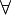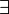# Difference between revisions of "12-240/Classnotes for Tuesday September 25"

Today's class dealt with the properties of vector spaces.

## Definition

Let F be a field. A vector space V over F is a set V of vectors with special element O ( of V) and two operations: (+): VxV->V, (.): FxV->V

VxV={(v,w): v,w$\in\!\,$ V}

FxV={(c,v): c$\in\!\,$ F, v$\in\!\,$ V}

Then, (+): VxV->V is (v,w)= v+w; (.): FxV->V is (c,v)=cv

Such that

VS1$\forall\!\,$ x, y$\in\!\,$ V: x+y = y+x

VS2$\forall\!\,$ x, y, z$\in\!\,$ V: x+(y+z) = (x+y)+z

VS3$\forall\!\,$ x$\in\!\,$ V: 0 ( of V) +x = x

VS4$\forall\!\,$ x$\in\!\,$ V,$\exists \!\,$ V$\in\!\,$ V: v + x= 0 ( of V)

VS5$\forall\!\,$ x$\in\!\,$ V, 1 (of F) .x = x

VS6$\forall\!\,$ a, b$\in\!\,$ F,$\forall\!\,$ x$\in\!\,$ V: (ab)x = a(bx)

VS7$\forall\!\,$ a$\in\!\,$ F,$\forall\!\,$ x, y$\in\!\,$ V: a(x + y)= ax + ay

VS8$\forall\!\,$ a, b$\in\!\,$ F,$\forall\!\,$ x$\in\!\,$ V: (a + b)x = ax + bx

## Polynomials

Definition : Pn(F) = {all polynomials of degree less than or equal n with coefficients in F}

                        = {anx^n + an-1x^n-1 + ... + a1x^1 + a1x^1}


0 = 0x^n + 0x^n-1 +...+ 0x^0

addition and multiplication: as you imagine

P(f) = {all polynomials with coefficients in F}

Take F= Z/2 |F| = 2

|P(F)| = infinite

in Pn(Z/2) x^3≠x^2

                  x^3 = 1*x^3+0x^2+0x+O = f
x^2 = 1*x^2+0x+0 = g
yet f(0)= g(0) and f(1)=g(1)


## Theorem

1. Cancellation Laws

  (a) x+z=y+z         ==> x=y
(b) ax+ay,a≠0       ==> x=y
(c) x≠0 of V, ax=bx ==> a=b


2. 0 of V is unique

3. Negatives are unique (so subtraction makes sense

4.(0 of F)x = 0 of V

5. a*0=0

6. (-a)x= - (ax) = a(-x)

7. a*v=0 <==> a=0 or v=0

## Proof

1. (a) x+z=y+z

       Find a w s.t. z+w=0 (V.S. 4)
(x+z)+w = (y+z)+w
Use VS2
x+(z+w) = y +(z+w)
x + 0   = y + o
Use VS3      x=y